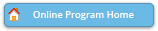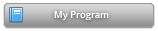#### Abstract Details

 Activity Number: 313 - Recent Developments in Statistical Inference Using Distance Correlation and Related Dependence Metrics Type: Invited Date/Time: Tuesday, July 30, 2019 : 10:30 AM to 12:20 PM Sponsor: Section on Nonparametric Statistics Abstract #300034 Title: Dependence Measures in Metric Spaces: From Distance Correlation to Earth Mover's Correlation Author(s): Gabor Szekely* Companies: NSF Keywords: distance correlation; Hilbert space; isometry; Wasserstein metric; dependence measure; axiom Abstract: Distance correlation was introduced by the speaker more than ten years ago for random variables taking values in Euclidean spaces. Russel Lyons generalized distance correlation to random variables in separable Hilbert spaces. If a metric space can be embedded into a Hilbert space isometrically, we can still apply distance correlation that satisfies all four axioms of a good dependence measure (see T.F. Mori and G.J. Szekely: Four simple axioms of dependence measures, Metrika 82, 1-16, 2019). We can always replace the metric space with its suitable homeomorphic image in a Hilbert cube. Then we can focus on “closeness” rather than on “distance” which is still enough for testing independence but we lose many good properties of distance correlation. How can we construct a dependence measure that satisfies the four axioms of dependence measures in all metric spaces? We can solve this problem by introducing the earth mover’s correlation. It applies to general metric spaces and satisfies our axioms. The computational complexity of the empirical earth mover’s correlation, however, is higher than that of distance correlation.

Authors who are presenting talks have a * after their name.